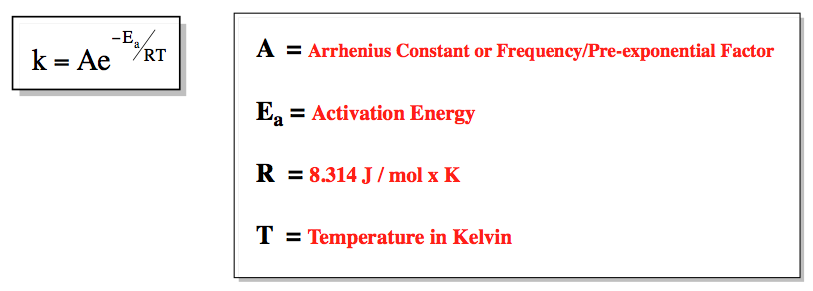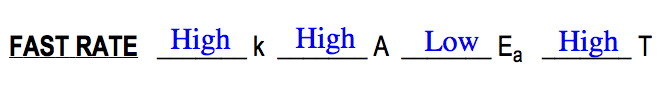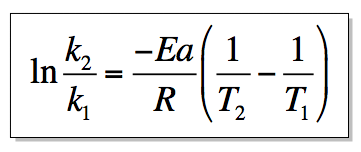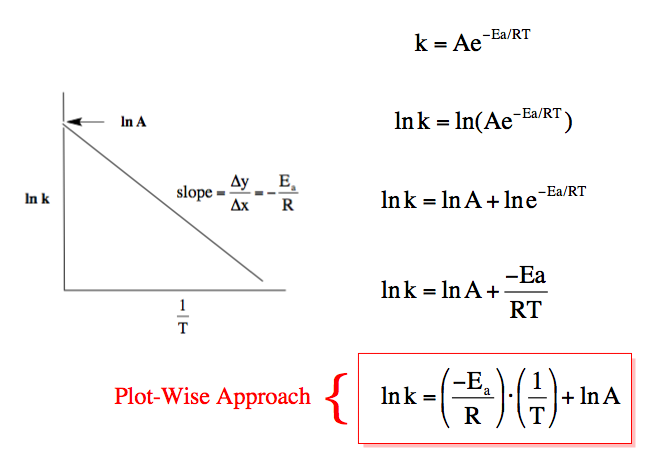Ch.13 - Chemical KineticsWorksheetSee all chapters
 Ch.1 - Intro to General Chemistry 2hrs & 53mins 0% complete Worksheet Ch.2 - Atoms & Elements 2hrs & 49mins 0% complete Worksheet Ch.3 - Chemical Reactions 3hrs & 25mins 0% complete Worksheet BONUS: Lab Techniques and Procedures 1hr & 38mins 0% complete Worksheet BONUS: Mathematical Operations and Functions 47mins 0% complete Worksheet Ch.4 - Chemical Quantities & Aqueous Reactions 3hrs & 30mins 0% complete Worksheet Ch.5 - Gases 3hrs & 47mins 0% complete Worksheet Ch.6 - Thermochemistry 2hrs & 28mins 0% complete Worksheet Ch.7 - Quantum Mechanics 2hrs & 35mins 0% complete Worksheet Ch.8 - Periodic Properties of the Elements 1hr & 57mins 0% complete Worksheet Ch.9 - Bonding & Molecular Structure 2hrs & 5mins 0% complete Worksheet Ch.10 - Molecular Shapes & Valence Bond Theory 1hr & 31mins 0% complete Worksheet Ch.11 - Liquids, Solids & Intermolecular Forces 3hrs & 40mins 0% complete Worksheet Ch.12 - Solutions 2hrs & 17mins 0% complete Worksheet Ch.13 - Chemical Kinetics 2hrs & 22mins 0% complete Worksheet Ch.14 - Chemical Equilibrium 2hrs & 26mins 0% complete Worksheet Ch.15 - Acid and Base Equilibrium 4hrs & 42mins 0% complete Worksheet Ch.16 - Aqueous Equilibrium 3hrs & 48mins 0% complete Worksheet Ch. 17 - Chemical Thermodynamics 1hr & 44mins 0% complete Worksheet Ch.18 - Electrochemistry 2hrs & 58mins 0% complete Worksheet Ch.19 - Nuclear Chemistry 1hr & 33mins 0% complete Worksheet Ch.20 - Organic Chemistry 3hrs 0% complete Worksheet Ch.22 - Chemistry of the Nonmetals 2hrs & 1min 0% complete Worksheet Ch.23 - Transition Metals and Coordination Compounds 1hr & 54mins 0% complete Worksheet

# Arrhenius Equation

See all sections
Sections
Rate of Reaction
Average Rate of Reaction
Arrhenius Equation
Rate Law
Integrated Rate Law
Collision Theory

The Arrhenius Equation illustrates the temperature dependence of the rate constant k.

###### Temperature and Rate

Temperature is one of the four factors that directly affects the rate of a reaction. It affects the rate of a reaction by impacting the rate constant k.

Concept #1: The Arrhenius Equation

Transcript

Hey guys! In this new video, we're going to take a look and see what exactly is the effect that temperature has on the rate of a chemical reaction. What we should realize is that temperature has a huge and profound effect on the rate of a reaction because temperature has a direct effect on our rate constant, k.
In fact, we're going to say increasing the temperature or adding or increasing the amount of catalyst will increase our k. If we increase the rate constant k, then that will increase the rate. We're going to say here to show this, we use the Arrhenius equation. The Arrhenius equation is k equals A times e to the negative Ea over Rt. Here we’re going to say A is called our frequency factor. Ea is called our energy of activation or activation energy. Now R is our constant. Because we're using energy, here the constant is 8.314 joules over moles times k. Then temperature is t, but the temperature here is in Kelvins.
Recall, one of the factors for a reaction to occur is that molecules have to collide in order to react. Molecule A has to hit molecule B. We're going to say for a reaction to be successful, the molecules must collide with sufficient energy and with the correct orientation. They have to be going fast enough so that they hit each other with enough force, but also they have to hit the activation site so that they actually stick together.

The Arrhenius Equation shows the dependence of the rate constant on the pre-exponential factor, absolute temperature and activitation energy of the reaction.In terms of what makes for a fast rate we can relate all these variables together.Concept #2: Besides the general Arrhenius equation there are also the two-point form and the linear/graphical form.

Transcript

Now we're going to say here we can convert the Arrhenius equation so that it gets a logarithmic form, also called the graphical form sometimes. If we have a plot or graph of something and we're trying to relate the Arrhenius equation to it, we usually use this graphical form also known as the plot wise approach to the Arrhenius equation.
When do we use this particular form? This is called the two-point form of the Arrhenius equation. We use this form anytime have two k values or we have two temperatures. That’s the time we use this. We’re going to say the Arrhenius equation can be converted so it forms this plot-wise approach. This equation is equal to y equals mx plus b, y is ln k, m is negative Ea over R, 1 over t is x, and ln A is b.
How did we come up with this equation? If we take a look, what you want to do here is you want to do ln in front of both these. But we’re also going to do ln in front of this e. That's how we got the ln k here. When we do ln in front of A and in front of e, it’s going to become plus ln A here. This right here is inverse log. When you do natural log in front of it, it basically cancels them out so then it becomes Ea over Rt. You can actually separate out the t here where it becomes negative Ea over R times 1 over t.
By putting ln in front of both things, that's how we’re able to separate it into this plot-wise approach. We'll see how best to use this later on. Realize that this equation here relates to this graph here. Remember, every time we plot something, it's y versus x. My y is ln k. My x here is 1 over t. Ea over R is m which is my slope. Remember, slope is just the change in y over the change in x. It's in this way that we can find the energy of activation. Later on when we do calculations, we'll see how best to use this type of equation.

When dealing with TWO rate constants or TWO temperatures then we use the Two-Point Form of the Arrhenius Equation.In order to relate the plot of a graph to the Arrhenius equation then we manipulate it into the plot-wise approach of the equation.Example #1: The reaction 2 HCl (g)  →  H2 (g) + Cl2 (g)  has E of 1.77 x 104 kJ/mol and a rate constant of 1.32 x 10-1  at 700 K. What is the rate constant at 685 K?

Practice: If a first order reaction has a frequency factor of 3.98 x 1013 s-1 and Ea of 160 kJ, then calculate the rate constant at 25oC.

Practice: Generally, the slower the rate of the reaction then the ___________ the energy of activation (Ea) and the higher the temperature, the ____________ the value of rate constant, k.